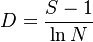# Margalef Index

## Definition

The Margalev Index (D) is a simple metric useful for the purpose of measuring diversity.

## Details

The index is only applicable for categorical data where all observations can be classified into a finite number of categories (species, types etc)

More precisely, if we have n observations values and a total of S categories, then the D index is defined as$D = \frac{S - 1}{\ln N}$

## Implementations

Open Source implementations of the Margalev index are available in